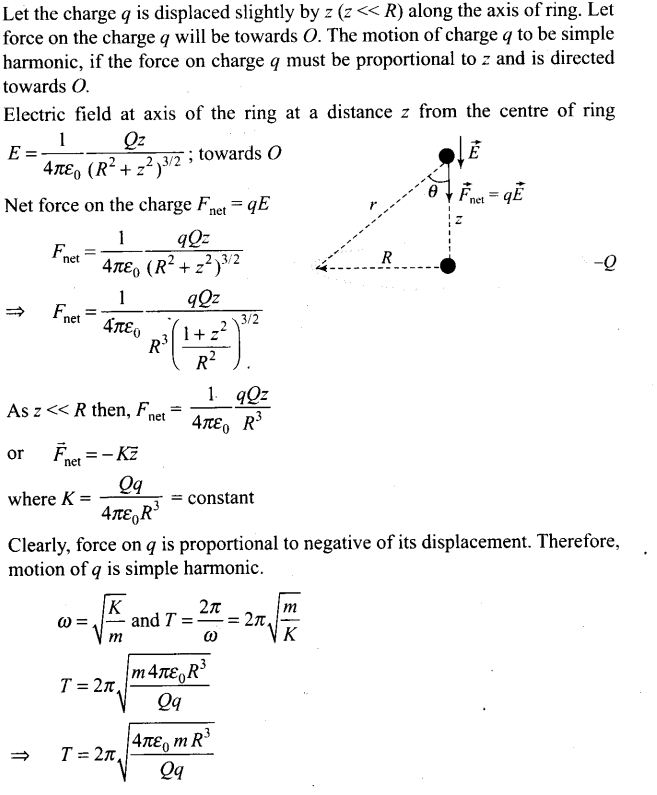# Electric Charges and Fields NCERT Exemplar Problems Solutions Physics

0
2067## NCERT Exemplar Problems Class 12 Physics Chapter 1 Electric Charges and Fields

Multiple Choice Questions

Question 1. In figure two positive charges q2 and q3 fixed along the y-axis, exert a net electric force in the +x-direction on a charge q1, fixed along the x-axis. If a positive charge Q is added at (x, 0), the force on q1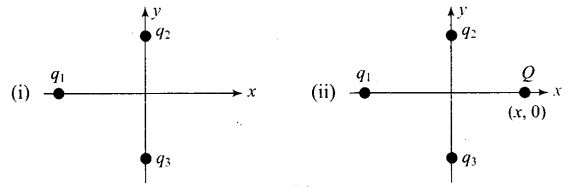(a) shall increase along the positive x-axis
(b) shall decrease along the positive x-axis
(c) shall point along the negative x-axis
(d) shall increase but the direction changes because of the intersection of Q with q2 and q3
Solution: (a)
Key concept: Total force acting on a given charge due to the number of charges is the vector sum of the individual forces acting on that charge due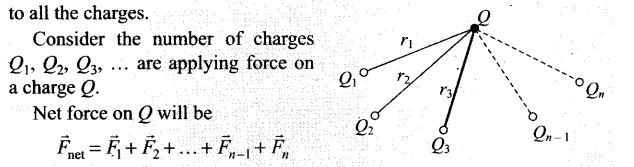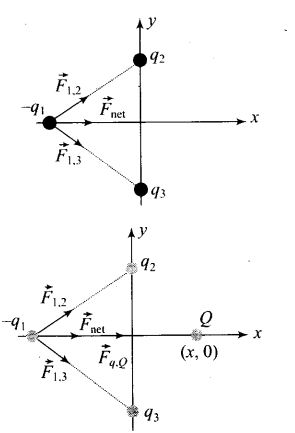We know that like charges repel and unlike charges attract. The net electrostatic force on the charge qx by the charges q2 and q3 is along the positive x-direction. Hence the nature of force between q1,q2 and q1, q3 should be attractive. It means qx should be negative. This can be represented by the figure given alongside:
Now a positive charge Q is placed at (x, 0), hence the nature of force between q1 and Q (positive) will be attractive and the force on q1 by the charge Q should be along positive x-axis direction. Now we can say that net force on the charge qx due to charges q2, q3 and Q should be along the same direction as given in the diagram alongside:
Now it is clear from the figure given above that the force on qx shall increase along the positive x-axis due to the presence of positive charge Q placed at (x, 0).

Question 2. A point positive charge is brought near an isolated conducting sphere (figure). The electric field is best given by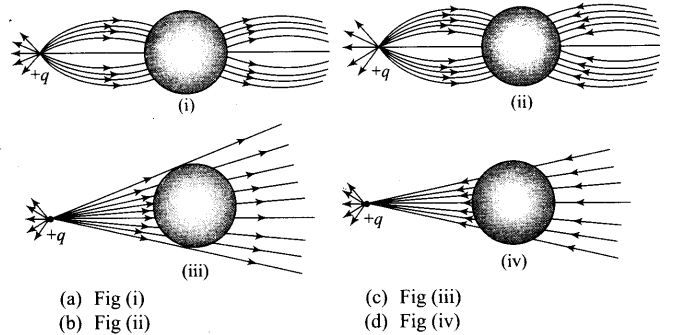Solution: (a)
Key concept:
• Electric field lines come out of positive charge and go into the negative charge.
• Tangent to the field line at any point gives the direction of the field at that point.
• Field lines are always normal to the conducting surface.
• Field lines do not exist inside a conductor.
The explanation to this problem-can be done by keeping two things in mind.
(i) Concept of induction
(ii) The electric field lines interact with a conducting body normally.
Let us discuss the phenomenon of induction involved in this case. When a positive point charge is brought near an isolated conducting sphere without touching the sphere, then the free electrons in the sphere are attracted towards the positive charge. Thus, the left surface of sphere has an excess of negative charge and the right surface of sphere has an excess of positive charge. It should be noted that both kinds of charges are bound in the metal sphere and cannot escape. They, therefore, reside on the surface of the sphere.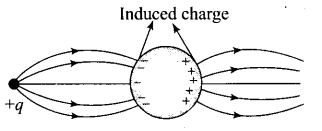An electric field lines start from a positive point charge and ends at negative charge induced on the left surface of sphere. Also, electric field line emerges from a positive charge, in case of single charge and ends at infinity.
Here, all these conditions are fulfilled in Fig. (i).

Question 3. The electric flux through the surface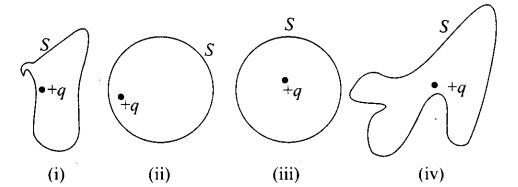(a) in Fig. (iv) is the largest
(b) in Fig. (iii) is the least
(c) in Fig. (ii) is same as Fig. (iii) but is smaller than Fig. (iv)
(d) is the same for all the figures
Solution: (d)
Key concept: According to Gauss’ law of electrostatics, the total electric flux out of a closed surface is equal to the charge enclosed divided by the permittivity,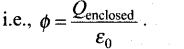Thus, electric flux through a surface doesn’t depend on the shape, size or area of a surface but it depends on the amount of charge enclosed by the surface.
In given figures the charge enclosed are same that means the electric flux through all the surfaces should be the same. Hence option (d) is correct.

Question 4. Five charges q1, q2, q3, q4 and q5 are fixed at their positions as shown in
figure, S is a Gaussian surface. The Gauss’ law is given by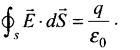Which of the following statements is correct?
(a) E on the LHS of the above equation will have a contribution from q,q5 and q1, q5 and q3 while q on the RHS will have a contribution from q1 and q4 only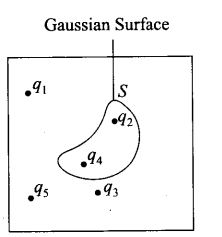(b) E on the LHS of the above equation will have a contribution from all charges while q on the RHS will have a contribution from q2 and q4 only
(c) E on the LHS of the above equation will have a contribution from all charges while q on the RHS will have a contribution from q1, q3 and q5 only
(d) Both E on the LHS and q on the RHS will have contributions from q2 and q4 only
Solution: (b)
Key concept: According to Gauss’ law, the term qenclosed on the right side of the equation Фs E . dS =qenclosed / ϵ0 includes the sum of all charges enclosed  by the surface called (Gaussian surface).
In left side equation, the electric field is due to all the charges present both inside as well as outside the Gaussian surface.
Hence in given question, E on LHS of the above equation will have a contribution from all charges while q on the RHS will have a contribution from q2 and q4 only. Hence option (b) is correct.

Question 5. Figure shows electric field lines in which an electric dipole P is placed as shown. Which of the following statements is correct?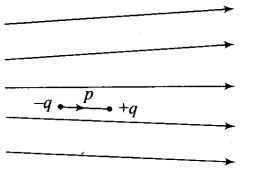(a) The dipole will not experience any force
(b)The dipole will experience a force towards right
(c)The dipole will experience a force towards left
(d)The dipole will experience a force upwards
Solution: (c)
Key concept: If the lines of forces are equidistant and parallel straight lines, the field is uniform and if either lines of force are not equidistant, or straight line or both, the field will be non-uniform. The number of electric field lmes passing per unit area is proportional to the strength of electric field. For example, see the following figures: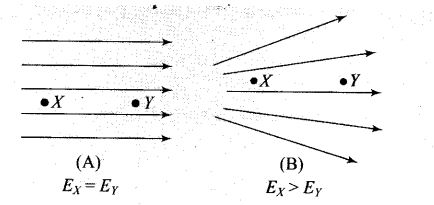Hence in given question, from given pattern of electric field lines it is clear that the strength of electric field decreases from left to right. As a result force on charges also decreases from left to right.
Here in given figure, the force on charge -q is greater than force on charge +q in turn dipole will experience a force towards left. Hence option (c) is correct.

Question 6. A point charge +q is placed at a distance d from an isolated conducting plane. The field at a point P on the other side of the plane is
(a) directed perpendicular to the plane and away from the plane
(b) directed perpendicular to the plane but towards the plane
(c) directed radially away from the point charge
(d) directed radially towards the point charge
Solution: (a) If a point positive charge is placed near an isolated conducting plane, free electrons are attracted towards the positive charge. Result of this some negative charge develops on the surface of the plane towards the positive charge side and an equal positive charge develops on opposite side of the plane. The electric field lines are away from positive charge and perpendicular to the surface. Hence the field at a point P on the other side of the plane is directed perpendicular to the plane and away from the plane, hence option (a) is correct.

Question 7. A hemisphere is uniformly charged positively. The electric field at a point on a diameter away from the centre is directed .
(a) perpendicular to the diameter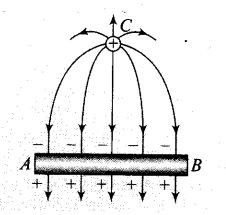(b) parallel to the diameter
(c) at an angle tilted towards the diameter
(d) at an angle tilted away from the diameter
Solution: (a) In case of a uniformly positive charged hemisphere, if a point situated at a point on a diameter away from the centre, the electric field should be perpendicular to the diameter. In this case the component of electric field intensity parallel to the diameter cancel out.

One or More than One Correct Answer Type

Question 8. If Фs E . dS = 0 over a surface, then
(a) the electric field inside the surface and on it is zero
(b) the electric field inside the surface is necessarily uniform
(c) the number of flux lines entering the surface must be equal to the number of flux lines leaving it
(d) all charges must necessarily be outside the surface
Solution: (c, d)
Key concept: We know electric flux is proportional to the number of electric field lines and the term Фs E . dS represents electric flux over the closed surface.
It means Фs E . dS represents the algebraic sum of number of flux lines entering the surface and number of flux lines leaving the surface.
If Фs E . dS = 0, this means the number of flux lines entering the surface must be equal to the number of flux lines leaving from it.
From Gauss’ law, we know Фs E . dS = q / ϵ0 , here q is the charge enclosed by , the closed surface. If Фs E . dS = 0 then q = 0, i.e., net charge enclosed by the surface must be zero.
Hence all other charges must necessarily be outside the surface. This is because of the fact that charges outside the surface do not contribute to the electric flux.

Question 9. The electric field at a point is
(a) always continuous
(b) continuous if there is no charge at that point
(c) discontinuous only if there is a negative charge at that point
(d) discontinuous if there is a charge at that point
Solution: (b, d) We cannot define electric field at the position of a charge, so we cannot say that electric field is always continuous. Hence option (a) is ruled out and option (d) is the correct choice. The electric field due to any charge will be continuous, if there is no other charge in the medium. It will be discontinuous if there is a charge at the point under consideration, hence option (b) is correct.

Question 10. If there were only one type of charge in the universe, then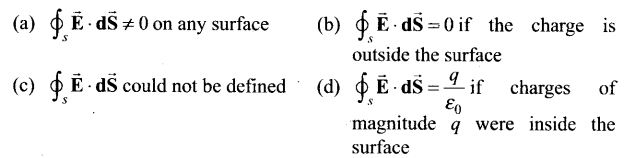Solution: (b, d) From Gauss’ law, we know Фs E . dS =q / ϵ0, here q is the charge
enclosed by the closed surface. If Фs E . dS= 0 then q = 0, i.e., net charge
enclosed by the surface must be zero.
If the charge is outside the surface, then charge enclosed by the surface is q = 0 and thus, (j) Фs E . dS = 0 . Hence options (b) and (d) are correct.

Question 11. Consider a region inside which there are various types of charges but the total charge is zero. At points outside the region,
(a) the electric field is necessarily zero
(b) the electric field is due to the dipole moment of the charge distribution only j
(c) the dominant electric field is ∝ 1/r3, for large r, where r is the distance from an origin in this regions r
(d) the work done to move a charged particle along a closed path, away from the region, will be zero
Solution: (c, d) From Gauss’ law, we know Фs E . dS =qenclosed / ϵ0. in left side equation.
the electric field is due to all the charges present both inside as well as outside the Gaussian surface. Hence if qenclosed= 0, it cannot be said that the electric field is necessarily zero. .
If there are various types of charges in a region and total charge is zero, the region may be supposed to contain a number of electric dipoles.
Therefore, at points outside the region (may be anywhere w.r.t. electric
dipoles), the dominant electric field ∝ 1/r3 for large r.
The electric field is conservative, work done to move a charged particle along a closed path, away from the region will be zero.

Question 12. Refer to the arrangement of charges in figure and a Gaussian surface of radius R with Q at the centre. Then,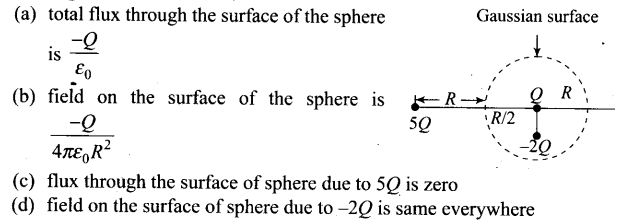Solution: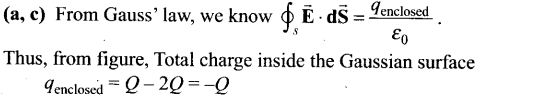The charge 5 Q lies outside the surface, thus it makes no contribution to electric flux through the given surface. Hence options (a) and (c) are correct.

Question 13. A positive charge Q is uniformly distributed along a circular ring of radius R. A small test charge q is placed at the centre of the ring figure. Then,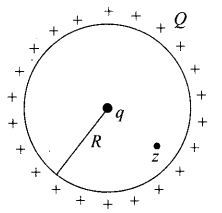(a) if q > 0 and is displaced away from the centre in the plane of the ring, it will be pushed back towards the centre
(b) if q < 0 and is displaced away from the centre in the plane of the ring, it will never return to the centre and will continue moving till it hits the ring
(c) if q < 0, it will perform SHM for small displacement along the axis
(d) q at the centre of the ring is in an unstable equilibrium within the plane of the ring for q > 0
Solution: (a, b, c, d) The positive charge Q is uniformly distributed along the circular ring then electric field at the centre of ring will be zero, hence no force is experienced by the charge if it is placed at the centre of the ring.
Now the charge is displaced away from the centre in the plane of the ring. There will be net electric field opposite to displacement will push back the charge towards the centre of the ring if the charge is positive. If charge is negative, it will experience net force in the direction of displacement and the charge will continue moving till it hits the ring. Also this negative charge is in an unstable equilibrium. Hence options (a), (b) and (d) are correct.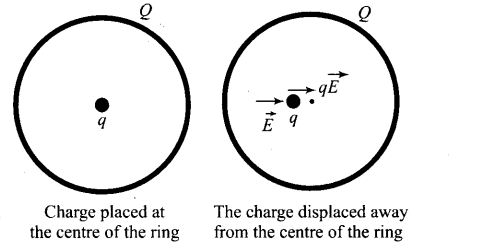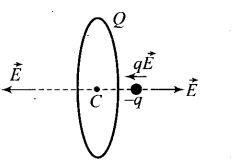The direction of electric field on the axis of a positively charged ring is along the axis of the ring and away from the centre of ring. If a negative charge is shifted away from the centre along the axis of ring, charge will experience a net force towards the centre and return to the centre and will perform SHM for small displacement along the axis.

Question 14. An arbitrary surface encloses a dipole. What is the electric flux through this surface?
Solution: Zero.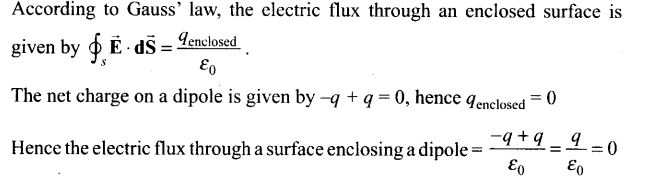Question 15. A metallic spherical shell has an inner radius R1 and outer radius R2. A charge Q is placed at the centre of the spherical cavity. What will be the surface charge density on .
(i) the inner surface (ii) the outer surface?
Solution: A charge Q is placed at the centre of the spherical cavity. So, the charge induced at the inner surface of the sphere will be -Q and at outer surface of the sphere is +Q.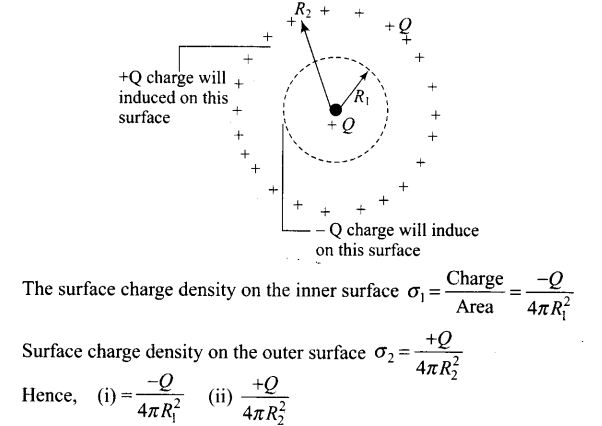Question 16. The dimensions of an atom are of the order of an Angstrom. Thus, there must be large electric fields between the protons and electrons. Why then is the electrostatic field inside a conductor zero?
Solution: In any neutral atom, the number of electrons and protons are equal, and the protons and electrons are bound into an atom with distinct and independent existence. Electrostatic fields are caused by the presence of excess charges. But there can be no excess charge on the intersurface of an isolated conductor. So, the electrostatic fields inside a conductor is zero despite the fact that the dimensions of an atom are of the order of an Angstrom.

Question 17. If the total charge enclosed by a Surface is zero, does it imply that the electric field everywhere on the surface is zero? Conversely, if the electric field everywhere on a surface is zero, does it imply that net charge inside is zero?
Solution: According to Gauss’ law, the flux associated with any closed surface is given
by Фs E . dS =qenclosed 0. The term qenclosed on the right side of the equation includes the sum of all charges enclosed by the surface called (Gaussian surface).
In left side equation,the electric field is due to all the charges present both inside as well as outside the Gaussian surface.
Thus, despite being total charge enclosed by a surface zero, it doesn’t imply that the electric field everywhere on the surface is zero, the field may be normal to the surface.
Also, conversely if the electric field everywhere on a surface is zero.

Question 18. Sketch the electric field lines for a uniformly charged hollow cylinder shown in figure.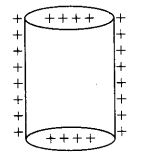Solution: The electric field lines starts from positive charges and move towards infinity and meet plane surface normally as shown in the figure below: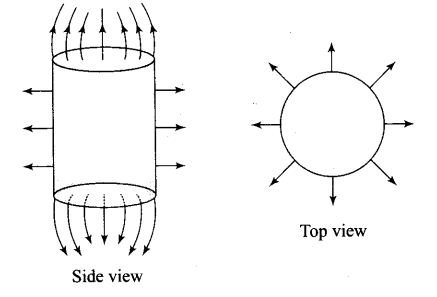Important point: No electric field lines will be present inside the cylinder because of electrostatic shielding. Electrostatic shielding/screening is the phenomenon of protecting a certain region of space from external electric field. Sensitive instruments and appliances are affected seriously with strong external electrostatic fields. Their working suffers and they may start misbehaving under the effect of unwanted fields.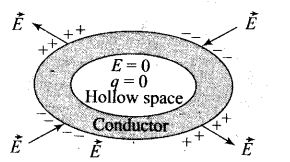The electrostatic shielding can be achieved by protecting and enclosing the sensitive instruments inside a hollow conductor because inside hollow conductors, electric fields is zero.

Question 19. What will be the total flux through the faces of the cube as given in the figure with side of length a if a charge q is placed at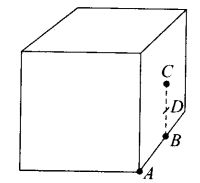(a) A a comer of the cube
(b) B mid-point of an edge of the cube (c) C centre of a face of the cube
(d) D mid-point of B and C
Solution: (a)Use of symmetry consideration may be useful in problems of flux calculation. We can imagine the charged particle is placed at the centre of a cube of side 2a. We can observe that the charge is being shared equally by
8 cubes. Therefore, total flux through the faces of the given cube =q/8ϵ0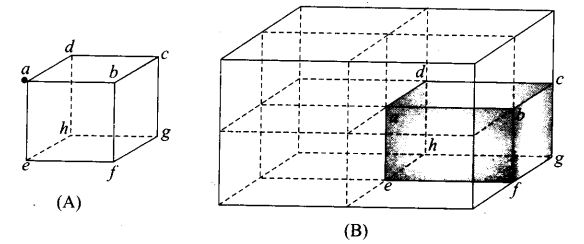(b) If the charge q is placed at B, middle point of an edge of the cube, it is being shared equally by 4 cubes. Therefore, total flux through the faces
of the given cube = q/4ϵ0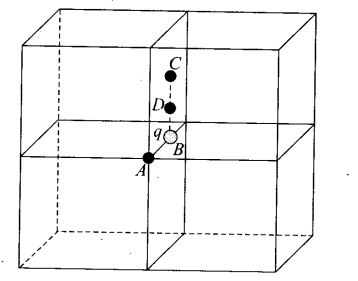(c) If the charge q is placed at C, the centre of a face of the cube, it is being shared equally by 2 cubes. Therefore, total flux through the faces of the
given cube =q/2ϵ0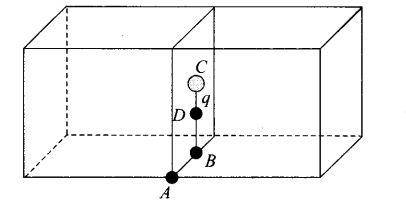(d) Finally, if charge q is placed at D, the mid-point of B and C, it is being shared equally by 2 cubes. Therefore, total flux through the faces of the
given cube =q/2ϵ0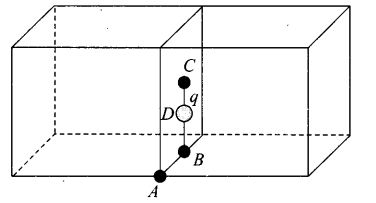Question 20. A paisa coin is made up of Al-Mg alloy and weight 0.75 g. It has a square shape and its diagonal measures 17 mm. It is electrically neutral and contains equal amounts of positive and negative charges.
Solution: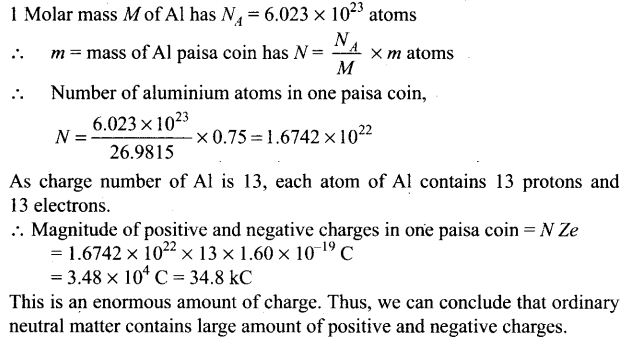Question 21. Consider a coin of Question 20. ft is electrically neutral and contains equal amounts of positive and negative charge of magnitude 34.8 kC. Suppose that these equal charges were concentrated in two point charges separated by
(i) 1 cm — (1/2  x diagonal of the one paisa coin)
(ii) 100 m (~ length of a long building)
(iii) 106 m (radius of the earth). Find the force on each such point charge in each of the three cases. What do you conclude from these results?
Solution: We know force between two point charges separated at a distance r,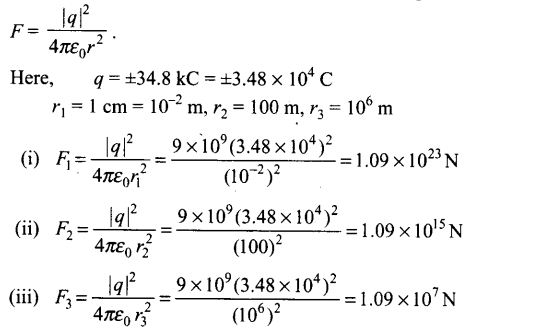Conclusion: Here we can observe that when positive and negative charges in ordinary neutral matter are separated as point charges, they exert very large force. It means, it is very difficult to disturb electrical neutrality of matter.

Question 22. Figure represents a crystal unit of cesium chloride, CsCl. The cesium atohis, represented by open circles are situated at the comers of a cube of side 0.40 nm, whereas a Cl atom is situated at
the centre of the Cube.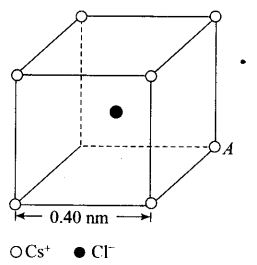The Cs atoms are deficient in one electron while the Cl atom carries an excess electron.
(i) What is the net electric field on the Cl atom due to eight Cs atoms?
(ii) Suppose that the Cs atom at the comer A is missing. What is the net force now on the Cl atom due to seven remaining Cs atoms?
Solution:
(i) The cesium atoms, are situated at the comers of a cube and Cl atom is situated at the centre of the cube. From the given figure, we can analyse that the chlorine atom is at equal distance from all the eight comers of cube where cesium atoms are placed. Thus, due to symmetry the electric field due to all Cs atoms, on Cl atom will cancel out. Hence net electric field at the centre of cube is zero.
(ii) We define force on a charge particle due to external electric field as F = qE. If eight cesium atoms, are situated at the comers of a cube, the net force on Cl atom is situated at the centre of the cube will be zero as net electric field at the centre of cube is zero. We can write that the vector sum of electric field due to charge A and electric field due to other seven charges at the centre of cube should be zero or, EA + Eseven charges= 0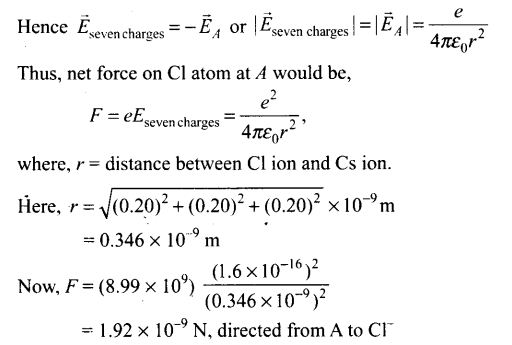Question 23. Two charges q and -3q are placed fixed on x-axis separated by a distance d. Where should a third charge 2q be placed such that it will not experience any force?
Solution: The force on any charge will be zero only if net electric field at the position of charge is zero. Let electric field is zero at a distance x from charge q.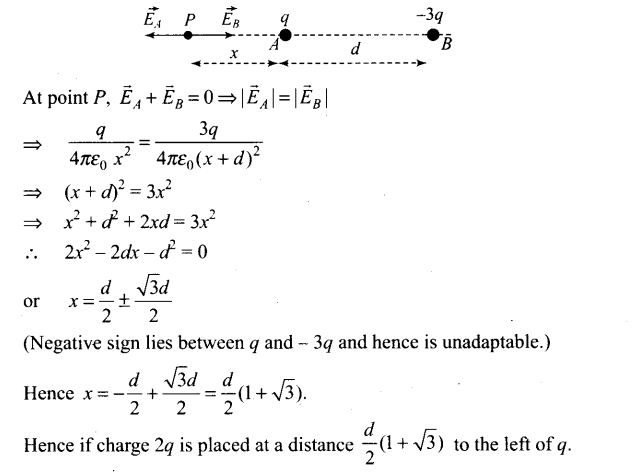Question 24. Figure shows the electric field lines around three point charges A, B and C.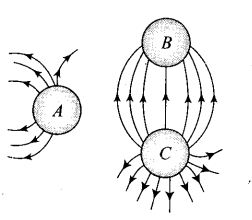(i) Which charges are positive?
(ii) Which charge has the largest magnitude? Why?
(iii) In which region or regions of the picture could the electric field be zero? Justify your answer.
(a) Near A (b) Near B
(c) Near C (d) Nowhere
Solution:
Key concept: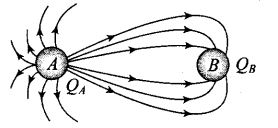• The number of lines originating or terminating on a charge is proportional to the magnitude of charge. In the following figure electric lines of force are originating from A and terminating at B, hence QA is positive while QB is negative. Also number of electric lines at force linked with QA are more than those linked with QB, hence |QA| > |QB|.
• The electric lines of forces always starts from a positive charge and ends at a negative charge. In case of a single isolated charge, electric lines of force start from positive charge ends at infinity.  There is no neutral point between unlike charges. Point between two
like charges where electrostatic force is zero is called neutral point. A neutral point may exist between two like charges. Also between two like charges the neutral point is closer to the charge with smaller magnitude.
(i) Here, in the figure, the electric lines of force starts from A and C.
Therefore, charges A and C must be positive.
(i) The number of electric lines of forces starting from charge C are maximum, so C must have the largest magnitude.
(iii) From the figure we see that a neutral point exists between charges A and C. Here, more number of electric lines of forces shows higher strength of charge C than A. Thus, electric field is zero near charge A hence neutral point lies near A.

Question 25. Five charges, q each are placed at the comers of a regular pentagon of side a.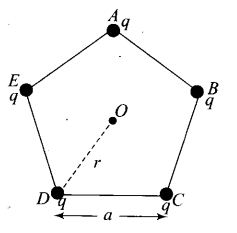(a) (i) What will be the electric field at O,
the centre of the pentagon?
(ii) What will be the electric field at O if the charge from one of the comers (say A) is removed?
(iii) What will be the electric field at O if the charge q at A is replaced by – q?
(b) How would your answer to (a) be affected if pentagon is replaced by n-sided regular polygon with charge q at each of its comers?
Solution: (a)
(i) The point O, the centre of the pentagon is equidistant from all the charges at the end point of pentagon. Thus, due to symmetry, the electric field due to all the charges are cancelled out. As a result electric field at O is zero.
(ii) We can write that the vector sum of electric field due to charge A and electric field due to other four charges at the centre of cube should be zero or,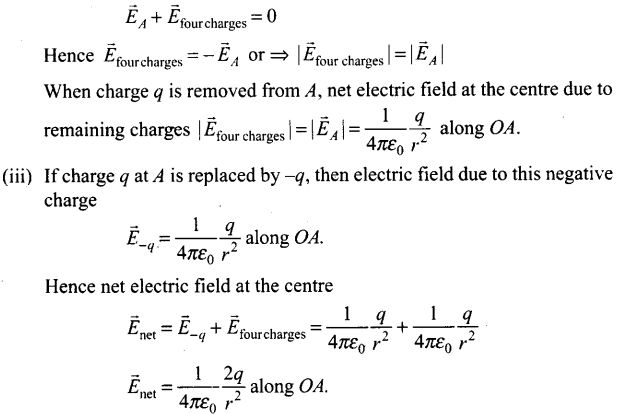(b) If pentagon is replaced by n-sided regular polygon with charge q at each of its comers. Here again charges are symmetrical about the centre. The net electric field at O would continue to be zero, it doesn’t depend on the number of sides or the number of charges.

Question 26. In 1959 Lyttleton and Bondi suggested that the expansion of the universe could be explained if matter carried a net charge. Suppose that the universe is made up of hydrogen atoms with a number density N, which is maintained a constant. Let the charge on the proton be ep = -(1 + y)e where e is the electronic charge.
(a) Find the critical value of y such that expansion may start.
(b) Show that the velocity of expansion is proportional to the distance from the centre.
Solution: (a) Let the Universe have a radius R. Assume that the hydrogen atoms are uniformly distributed. The expansion of the universe will start if the coulomb repulsion on a hydrogen atom, at R is larger than the gravitational attraction.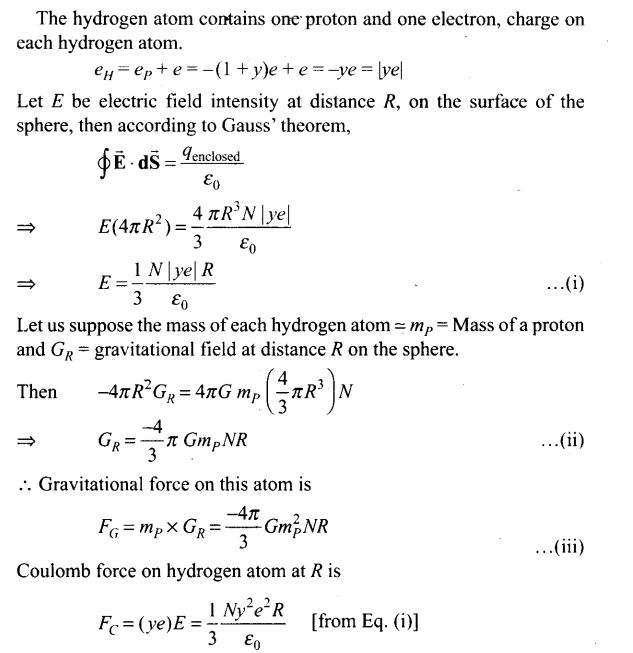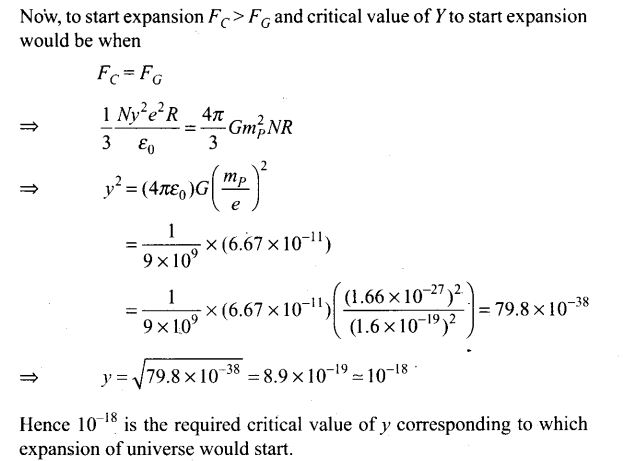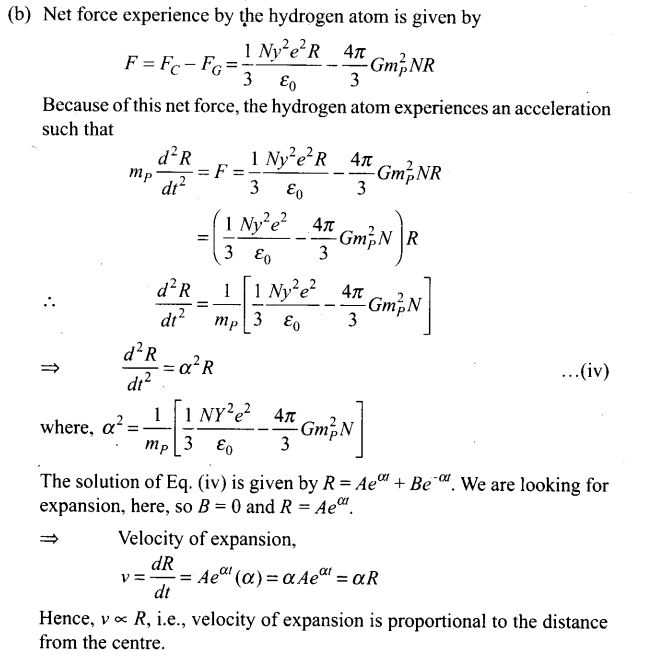Question 27. Consider a sphere of radius R with charge density distributed as p(r) = kr for r <= R
= 0 for r > R.
(a) Find the electric field at all points r.
(b) Suppose the total charge on the sphere is 2e where e is the electron charge. Where can two protons be embedded such that the force on each of them is zero. Assume that the introduction of the proton does not alter the negative charge distribution?
Solution: (a) The expression of charge density distribution in the sphere suggests that the electric field is radial.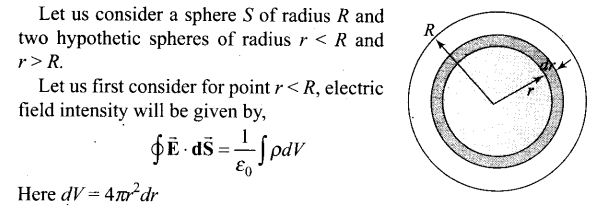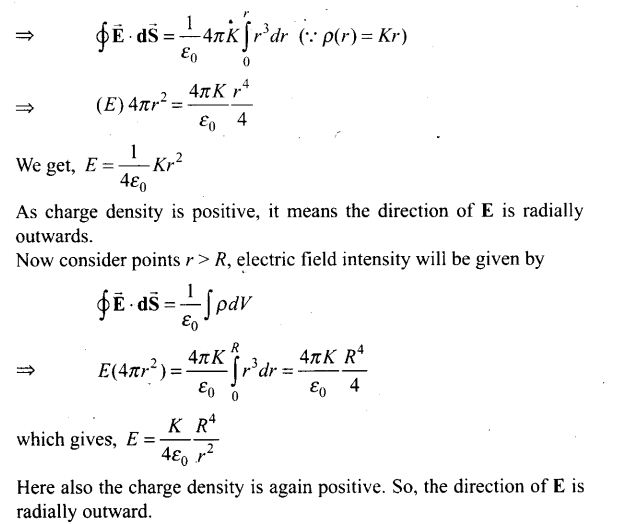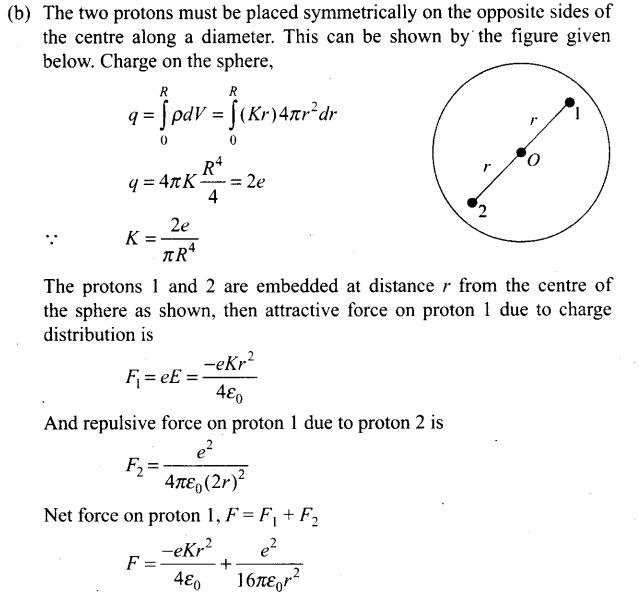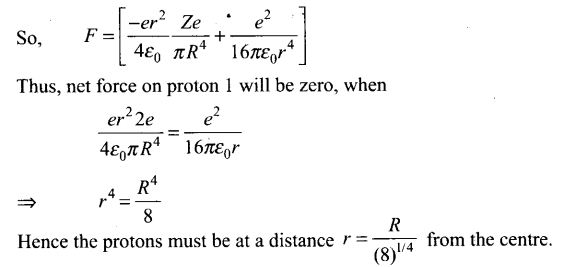Question 28.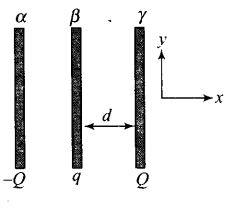Two fixed, identical conducting plates (a and P), each of surface area .S’ are charged to -Q and q, respectively, where Q > q >0. A third identical plate (j), free to move is located on the other side of the plate with charge q at a distance d (figure). The third plate is released and collides with the plate p. Assume the collision is elastic and the time of collision is sufficient to redistribute charge amongst p and y
(a) Find the electric field acting on the plate y before collision.
(b) Find the charges on P and yafter the collision.
(c) Find the velocity of the plate yafter the collision and at a distance d from the plate /?.
Solution: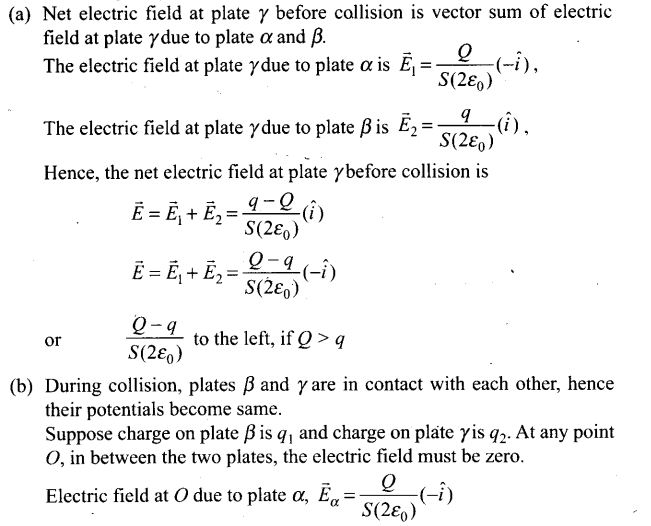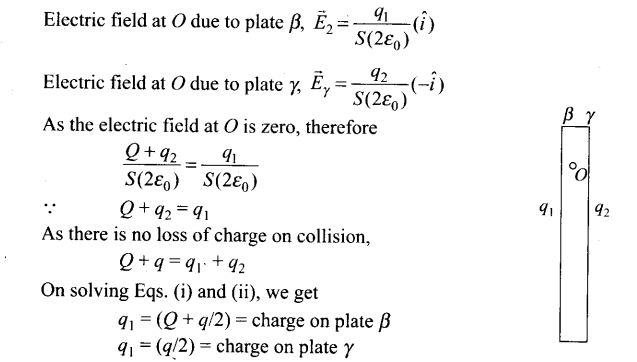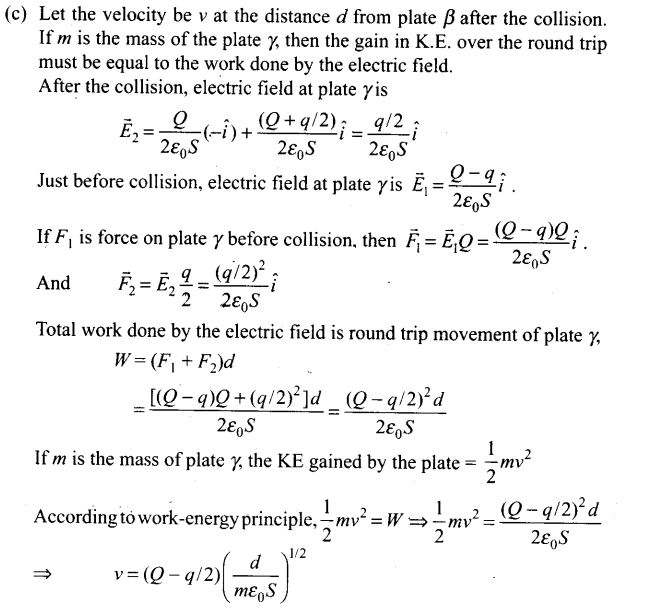Question 29. There is another useful system of units, besides the SI/MKS. A system, called the CGS (Centimeter-Gram-Second) system. In this system, Coulomb’s law
is given by F = (Q q/r2)r .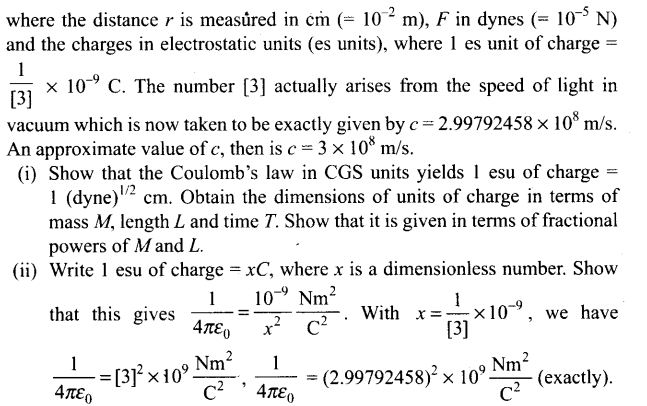Solution: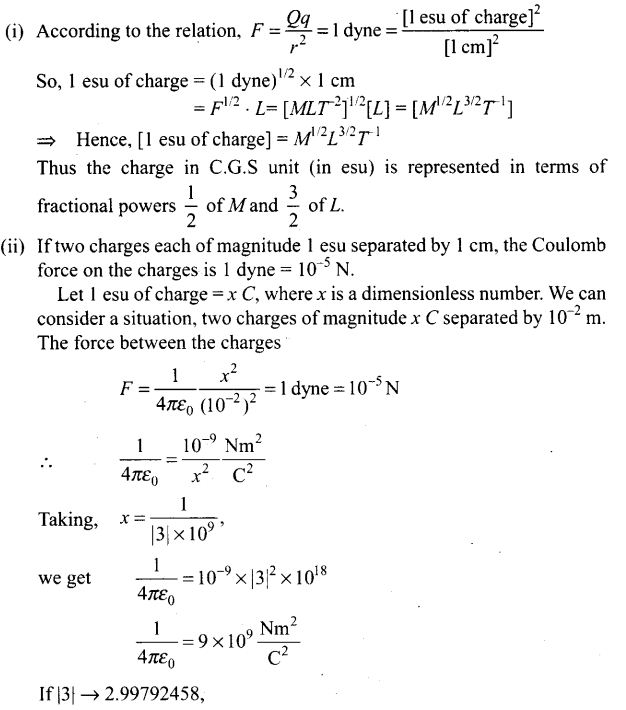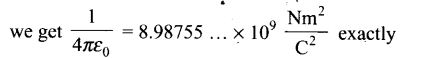Question 30.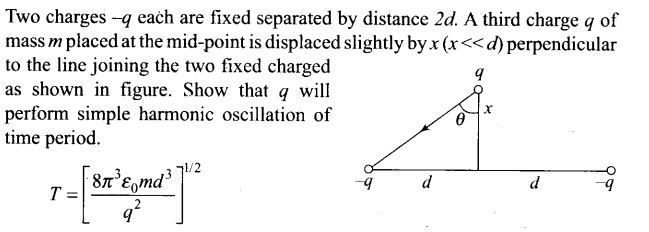Solution: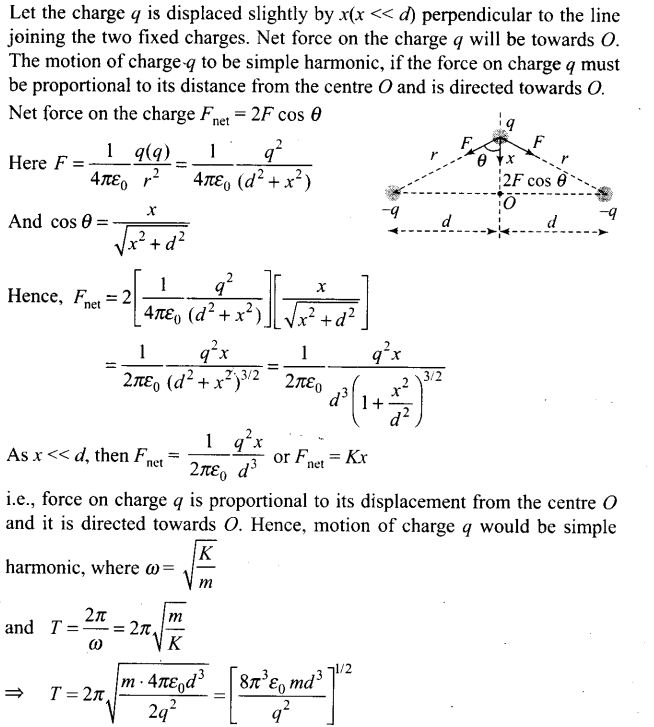Question 31. Total charge -Q is uniformly spread along the length of a ring of radius R. A small test charge +q of mass m is kept at the centre of the ring and is given a gentle push along the axis of the ring.
(a) Show that the particle executes a simple harmonic oscillation.
(b) Obtain its time period.
Solution: